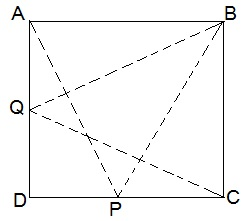Guru

# P and Q are any two points lying on the sides DC and AD respectively of a parallelogram ABCD. Show that ar(APB) = ar(BQC). Q.3

• 0

Hello sir i want to know the best solution of the question from exercise 9.2of math of Areas of Parallelograms and Triangles chapter of class 9th give me the best and easy for solving this question how i solve it of question no.3 P and Q are any two points lying on the sides DC and AD respectively of a parallelogram ABCD. Show that ar(APB) = ar(BQC).

Share

1.ΔAPB and parallelogram ABCD lie on the same base AB and in-between same parallel AB and DC.

ar(ΔAPB) = ½ ar(parallelogram ABCD) — (i)

Similarly,

ar(ΔBQC) = ½ ar(parallelogram ABCD) — (ii)

From (i) and (ii), we have

ar(ΔAPB) = ar(ΔBQC)

• 0